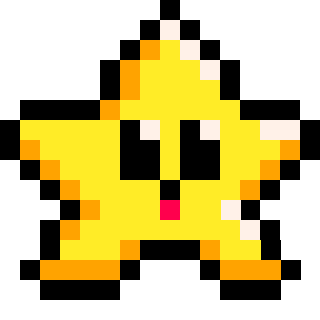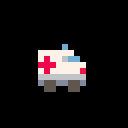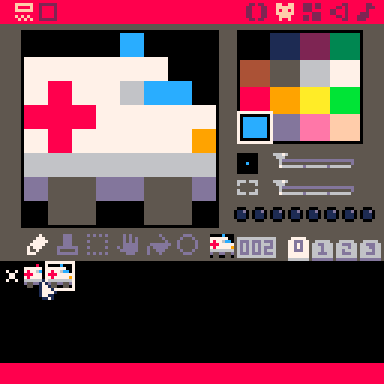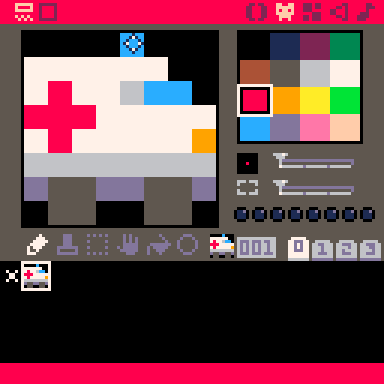# sprites

`sget` = "spritesheet get"

`` sget( x, y )``
 x the distance from the left side of the screen (in pixels). y the distance from the top side of the screen (in pixels).

This function will return the color number (0-15) of a single pixel currently drawn at the (x,y) coordinate specified on the sprite sheet. If you request a pixel that is outside of the screen, then `sget` will return 0 (zero).Example:

``````sset(10,20,8)              --draw at (10,20), a red pixel
pixel_color = sget(10,20)  --returns 8 (red)
``````578

3 Nov 2023

`sset` = "spritesheet set"

`` sset( x, y, [color] )``
 x the distance from the left side of the spritesheet (in pixels). y the distance from the top side of the spritesheet (in pixels). color (optional) a color number

This function will draw a single pixel to the (x,y) coordinate specified, but on the spritesheet not the screen. You can specify the color as a number (0-15) according to the palette. The default color is the current draw color (last color set by either `color()` function or by a drawing function's color argument).Example:

``````sset(10,0,8)    --draw at (10,0), a red pixel
sset(11,1)    --draw at (11,1), still a red pixel
spr(1,20,30)    --draw sprite 1 at (20,30)

color(12)       --set draw color to #12, blue
sset(16,0)      --draw at (16,0), a blue pixel
sset(17,1)      --draw at (17,1), a blue pixel
spr(2,20,38)    --draw sprite 2 at (20,38)``````

As you can see, you can specify the draw color multiple ways. In the first example we use the color argument in the `sset` function the first line and the same color is carried over to the second `sset`. In the second example, we set the color first with the `color` function which is also carried over to `sset`.### Simple Animations

This function can save you some sprite sheet space if you want to animate just one or two pixels, such as this flashing light on an ambulance.Here is how you could make this animation using 2 sprites. We use the sprite editor to manually show what the code is doing: changing between drawing sprite 1 and sprite 2.

``````cls()
car=1
counter=0

function _update()
counter+=.1
if counter<1 then
car=1
elseif counter<2 then
car=2
else
counter=0
end
end

function _draw()
spr(car,20,30)
end``````And here is how you can save a tile on the sprite sheet by doing the same animation with only 1 sprite and the `sset()` function. We use the sprite editor to manually show what the code is doing: swapping one pixel in the sprite sheet to a different color.

``````cls()
car=1
counter=0

function _update()
counter+=.1
if counter<1 then
sset(12,0,8)
elseif counter<2 then
sset(12,0,12)
else
counter=0
end
end

function _draw()
spr(car,20,30)
end
``````So if your animations are as simple as swapping a couple colored pixels around, consider using this function to help be more efficient with your sprite sheet space.422

3 Nov 2023

fget

460

22 Jan 2023

fset

346

22 Jan 2023

spr

613

22 Jan 2023

sspr

571

22 Jan 2023

475

16 Apr 2023

`Font`